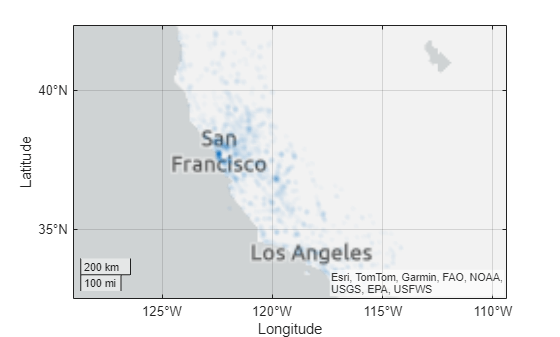Main Content

# geodensityplot

Geographic density plot

## Syntax

``geodensityplot(lat,lon)``
``geodensityplot(lat,lon,weights)``
``geodensityplot(___,Name,Value)``
``geodensityplot(gx,___)``
``dp = geodensityplot(___)``

## Description

example

````geodensityplot(lat,lon)` creates a density plot in a geographic axes from locations specified (in degrees) by the coordinate vectors `lat` and `lon`. `lat` and `lon` must be the same size.```
````geodensityplot(lat,lon,weights)` specifies weights for the data points in `weights`.```
````geodensityplot(___,Name,Value)` specifies `DensityPlot` properties using one or more Name,Value pair arguments.```
````geodensityplot(gx,___)` plots in the geographic axes specified by `gx` instead of the current axes.`gx`.```
````dp = geodensityplot(___)` returns a `DensityPlot` object.```

## Examples

collapse all

Set up latitude and longitude data.

```lon = linspace(-170,170,3000) + 10*rand(1,3000); lat = 50 * cosd(3*lon) + 10*rand(size(lon));```

Specify weights for each data point.

`weights = 101 + 100*(sind(2*lon));`

Create the geographic density plot, specifying the colors used with the plot.

`geodensityplot(lat,lon,weights,'FaceColor','interp')`## Input Arguments

collapse all

Latitude coordinates of data points, specified as a real, numeric, finite vector in degrees, within the range [-90 90]. The vector can contain embedded `NaN`s. `lat` must be the same size as `lon`.

Example: `[43.0327 38.8921 44.0435]`

Data Types: `single` | `double`

Longitude coordinates in degrees, specified as a real, numeric, finite vector. The vector can contain embedded `NaN`s. `lon` must be the same size as `lat`.

Longitudes must span less than (or equal to) 360 degrees.

Example: `[-107.5556 -77.0269 -72.5565]`

Data Types: `single` | `double`

Weights assigned to data, specified as an empty array, a scalar, or a numeric array. If you specify a numeric vector, the vector must be the same length as the `lat` and `lon` vectors. The `weights` vector is typically additional data you have related to the location data in `lat` and `lon`.

Data Types: `single` | `double` | `int8` | `int16` | `int32` | `int64` | `uint8` | `uint16` | `uint32` | `uint64`

Target geographic axes, specified as a `GeographicAxes` object. You can modify the appearance and behavior of a `GeographicAxes` object by setting its properties. For a list of properties, see GeographicAxes Properties.

### Name-Value Pair Arguments

Specify optional comma-separated pairs of `Name,Value` arguments. `Name` is the argument name and `Value` is the corresponding value. `Name` must appear inside quotes. You can specify several name and value pair arguments in any order as `Name1,Value1,...,NameN,ValueN`.

Example: ```dp = geodensityplot(lat,lon,weights,'FaceColor','g')```

Note

The density plot properties listed here are frequently used properties. For a complete list, see DensityPlot Properties.

Face transparency, specified as one of these values:

• Scalar in range `[0,1]` — Use uniform transparency across all the faces. A value of `1` is fully opaque and `0` is completely transparent. Values between `0` and `1` are semitransparent.

• `'interp'` — Use interpolated transparency for each face. The transparency varies across each face by interpolating the values at the vertices. Transparency values are chosen from the parent axes alphamap.

Face color, specified as one of the values in this table.

ValueDescription
`'interp'`

Use interpolated coloring based on density values. Colors are chosen from the parent axes colormap.

RGB triplet

Three-element row vector whose elements specify the intensities of the red, green, and blue components of the color. The intensities must be in the range `[0,1]`, for example, `[0.4 0.6 0.7]`.

`ColorSpec`

MATLAB® ```ColorSpec (Color Specification)```. You can specify some common colors by name.

Radius of influence each point has on density calculation, specified as a numeric scalar. When used in a `GeographicAxes`, the value is measured in meters.

## Output Arguments

collapse all

Geographic density plot, returned as a `DensityPlot` object.

## Tips

• To customize the geographic axes, modify geographic axes properties. For a list of properties, see GeographicAxes Properties.

• if you have Mapping Toolbox™, you can specify basemaps of your own choosing using the `addCustomBasemap` function.

• When you plot on geographic axes, the `geodensityplot` function assumes that coordinates are referenced to the WGS84 coordinate reference system. If you plot using coordinates that are referenced to a different coordinate reference system, then the coordinates may appear misaligned.

## See Also

### Topics

Introduced in R2018b

 Alignment of boundaries and region labels are a presentation of the feature provided by the data vendors and do not imply endorsement by MathWorks®.

Download ebook